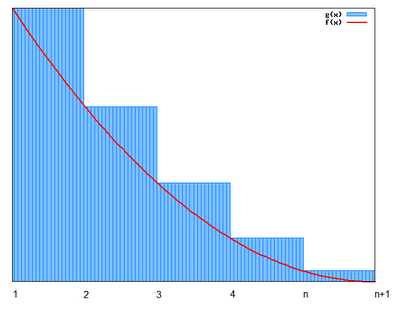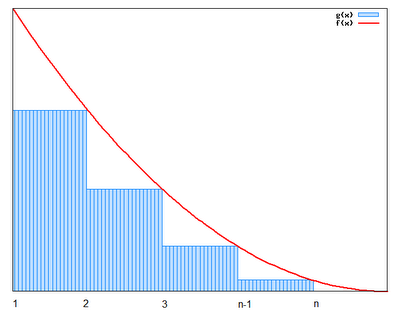## Saturday, November 11, 2006

### lim (s ↓ 1) (s-1)ζ(s) = 1

Before beginning the derivation of Kummer's class formula for cyclotomic integers, it is necessary to review one important property from the Riemann zeta function.

The content in today's blog is taken from Harold M. Edwards' Fermat's Last Theorem.

Theorem 1: lim(s ↓ 1) (s-1)ζ(s) = 1

Proof:

(1) From a previous result (see Theorem 4, here), we know that:

ζ(s) = ∑ (1/ns) = ∏ (1 - p-s)-1

and that:

for s greater than 1, both sides of this equation are absolutely convergent (see Theorem 1 and Theorem 2, here).

(2) Using the Integral Test (see Theorem 1, here),

We know that if ∑ (1/ns) converges, then ∫ (1,∞) 1/xsdx also converges.

(3) If we define a function so that f(x) = 1/ceiling(x)s, we can conclude that ∫ (1,∞) 1/xs is less than ∫ (1,∞) 1/ceiling(x)s = ∑ (n=1,∞) 1/ns which is less than 1 + ∫ (1,∞) 1/xsdx since:

(a) ∫ (1,∞) 1/xs is less than ∫ (1,∞) 1/ceiling(x)s

At each point x ≤ ceiling(x) so that at each point xs ≤ ceiling(x)s.(b) ∫ (1,∞) 1/ceiling(x)s = ∑ (n=1,∞) 1/ns

This follows from the fact that for each interval, ∫ (x,x+1) 1/ceiling(x)s= ∑(n=x,x) 1/ns.

(c) ∑ (n=1,∞) 1/ns is less than 1 + ∫ (1,∞) 1/xsdx

This follows since ∑ (n=1,∞) 1/ns - 1 is less than ∫ (1,∞) 1/xsdx and therefore ∑ (n=1,∞) 1/ns is less than 1 + ∫ (1,∞) 1/xsdx.(4) ∫ (1,∞) 1/xsdx = 1/(s-1)

(a) ∫ (1,∞) 1/xsdx = lim (t → ∞) ∫ (1,t) 1/tsdt

(b) ∫ 1/tsdt = ∫ t-s dt = 1/(-s+1) (t-s+1) + C = -1/(s-1)(1/ts-1) + C [See here for review of integral if needed]

(c) lim (t → ∞) ∫ (1,t) 1/tsdt = lim(t → ∞) ( [-1/(s-1)][1/ts-1] + [1/(s-1)]*[1/1s-1])=

= lim (t → ∞) [(-1)/[(s-1)*(ts-1)] + [1/(s-1)]*1]

(d) As t → ∞, 1/t → 0 so we get:

= [0*(-1)/(s-1)] + [1/(s-1)] = 1/(s-1)

(5) This gives us that:

1/(s-1) is less than ζ(s) is less than 1 + 1/(s-1)

(6) Multiplying (s-1) to all sides, gives us:

1 is less than (s-1)*ζ(s) is less than (s-1) + 1 = s

(7) Now as s goes to 1, it is clear that we have:

1 ≤ (s-1)*ζ(s) ≤ 1.

(8) Using the Squeeze Law (see Lemma 3, here), we can conclude that the limit is 1.

QED

References